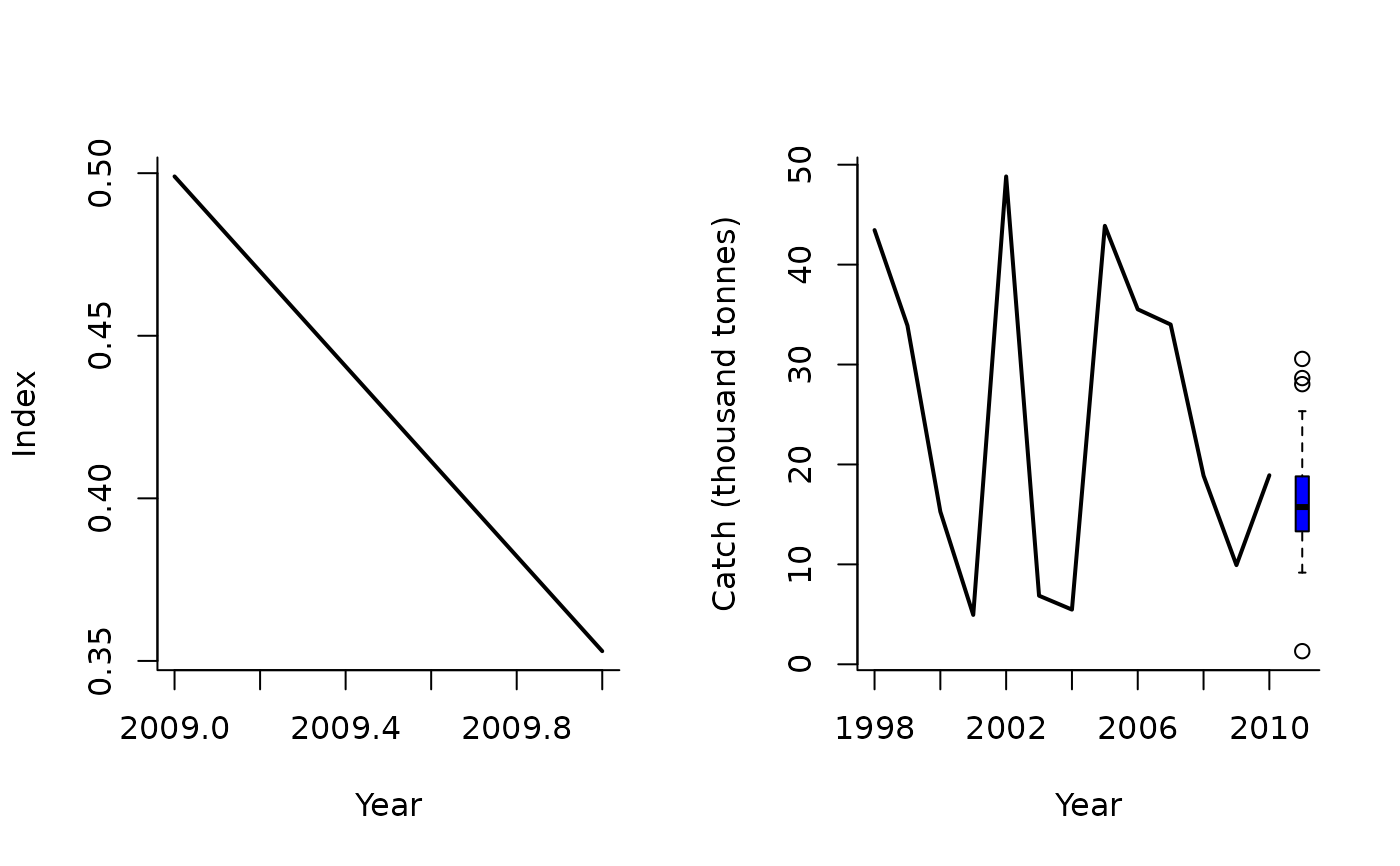An MP that makes incremental adjustments to TAC recommendations based on the apparent trend in surplus production. Based on the theory of Mark Maunder (IATTC)

SPmod(x, Data, reps = 100, plot = FALSE, alp = c(0.8, 1.2), bet = c(0.8, 1.2))

## Arguments

x

A position in the data object

Data

A data object

reps

The number of stochastic samples of the MP recommendation(s)

plot

Logical. Show the plot?

alp

Condition for modifying the TAC (bounds on change in abundance)

bet

Limits for how much the TAC can change among years

## Value

An object of class Rec-class with the TAC slot populated with a numeric vector of length reps

A numeric vector of TAC recommendations

## Details

Note that this isn't exactly what Mark has previously suggested and is stochastic in this implementation.

The TAC is calculated as: $$\textrm{TAC}_y = \left\{\begin{array}{ll} C_{y-1} \textrm{bet}_1 & \textrm{if } r < \alpha_1 \\ C_{y-1} & \textrm{if } \alpha_1 < r < \alpha_2 \\ \textrm{bet}_2 (b_2 - b_1 + C_{y-2} ) & \textrm{if } r > \alpha_2 \\ \end{array}\right.$$ where $$\textrm{bet}_1$$ and $$\textrm{bet}_2$$ are elements in bet, $$r$$ is the ratio of the index in the most recent two years, $$C_{y-1}$$ is catch in the previous year, $$b_1$$ and $$b_2$$ are ratio of index in $$y-2$$ and $$y-1$$ over the estimate of catchability $$\left(\frac{I}{A}\right)$$, and $$\alpha_1$$, $$\alpha_2$$, and $$\alpha_3$$ are specified in argument alp.

## Required Data

See Data-class for information on the Data object

SPmod: Cat, Ind

## Rendered Equations

See Online Documentation for correctly rendered equations

Other Surplus production MPs: Fadapt(), Rcontrol(), SPMSY(), SPSRA(), SPslope()

T. Carruthers

## Examples

SPmod(1, Data=MSEtool::Atlantic_mackerel, plot=TRUE)#> TAC (median)
#>      15.1027